Contrast Stretching

The contrast of an image is the distribution of its dark and light pixels . An image of low contrast has a small difference between its dark and light pixel values. The histogram of a low contrast image is usually skewed either to the left (mostly light), to the right (mostly dark), or located around the right (mostly gray). Figure 3.1 shows histograms of low contrast images. Contrast stretching is a technique used to stretch the histogram of an image so that the full dynamic range of the image is filled.

Listing 3.1 shows one of the two most popular contrast stretching techniques, basic contrast stretching. It computes the highest and the lowest pixel intensity values, sets them to 255 and 0 respectively, and scales all other pixel intensities according. This technique works best when the histogram of the image is Gaussian.

Listing 3.2 shows another popular contrast stretching technique, end-in-search. It requires a lower threshold and a higher threshold as inputs. The lower threshold, low, is the percentage of pixels to be set to 0. The higher threshold, high, is the percentage of pixels to be set to 255. The output value is calculated according to the formula below :

 0 for x <= low output (x) = 255 * (x-low)/(high-low) for low <= x<= high 255 for high <= x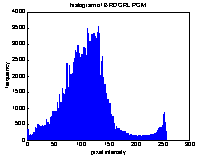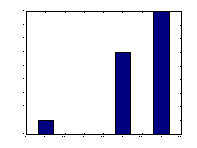Figure 3.1 Histograms of low-contrast images
NAME

auto_contrast - performs the basic contrast stretching of an input image.

SYNPOSIS

auto_contrast(input_file_name, output_file_name)

DESCRIPTION

auto_contrast performs the basic contrast stretching operation of an input image. It reads an image from the input file, computes the image's highest and lowest pixel values, sets them to 255 and 0 respectively, and scales other intensities according. New pixel values are calculated using the formula below:

New_value=[(Old_value-Min_value)/(Max_value-Min_value)]*255

auto_contrast then transforms the original image to a new image and writes it to the output file. The input image can be in Windows Bitmap (bmp), Hierarchical Data Format (HDF), Joint Photographic Experts Group (JPEG), Windows Paintbrush (PCX), Tagged Image File Format (TIFF), or Window Dump (XWD) format. The output file is in TIFF format.

EXAMPLES

auto_contrast('ROOF.TIF', 'ac_rf.tif')

This example performs basic contrast stretching on the image ROOF (Figure 3.2)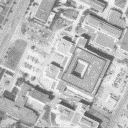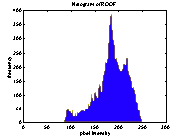a. Original ROOF image b. Histogram of ROOF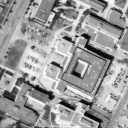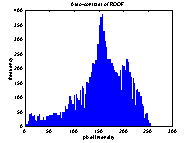c. Auto-contrast of ROOF d. Histogram of auto-contrasted ROOF

Figure 3.2 Auto_contrast stretching

NAME

ends_in - performs ends-in-search contrast stretching of an image.

SYNPOSIS

ends_in(input_file_name, output_file_name, low, high)

ends_in(input_file_name, output_file_name, [low, high])

DESCRIPTION

ends_in performs end-in-search contrast stretching of an image. It reads an image, computes its histogram, marches up the histogram until the lower threshold is reached and sets all pixel intensities below the lower threshold to 0. It then marches down the histogram until the higher threshold is reached and sets all pixel intensities above the higher threshold to 255. Next, it calculates the output values of other pixel intensities, transforms the input image, and writes the output image to the output file. The input image can be in Windows Bitmap (bmp), Hierarchical Data Format (HDF), Joint Photographic Experts Group (JPEG), Windows Paintbrush (PCX), Tagged Image File Format (TIFF), or Window Dump (XWD) format. The format of the output file is TIFF.

 low --the percentage of pixels to be set to 0 high --the percentage of pixels to be set to 255

EXAMPLES

ends_in('ROOF.TIF', 'ei_rf.tif', 1, 1)

This example performs end-in-search contrast stretching on ROOF. 1% of the pixels are

set to 0 and 1% of the pixels are set to 255 (Figure 3.3a)

ends_in('ROOF.TIF', 'ei_rf.tif', 5, 5)

This example performs end-in-search contrast stretching on ROOF. 5% of the pixels are set to 0 and 5% of the pixels are set to 255 (Figure 3.3b)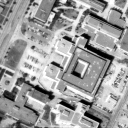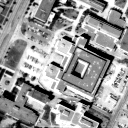a. End-in-search contrast stretched ROOF with 1% set to 1 and 1% set to 255 b. End-in-search contrast stretched ROOF with 5% set to 1 and 5% set to 255

Figure 3.3 Ends_in contrast stretching